# Comparison of Distribution Functions

DistributionFunctional FormMeanStandard Deviation

### Binomial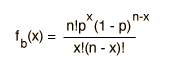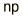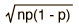### Gaussian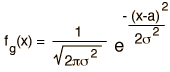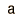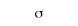### Poisson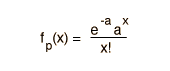If the probability of an event is p = and there are n = events, then the probability of that event being observed is:
 Distribution Value of x Probability Binomial x 10^ Gaussian x 10^ Poisson x 10^
For these conditions, the mean number of events is and the standard deviation is .

### Note!

The binomial distribution is the exact probability, so the above comparison can serve to check on the conditions under which the Gaussian and Poisson distributions are good approximations to it. If you substitute numbers, you will find that the Poisson is a good approximation if the probability p is small and the number of events n is large. If the number of events is large and the probability is also sizable, then the Gaussian will be a better approximation.
Index

Distribution functions

 HyperPhysics*****HyperMath R Nave
Go Back